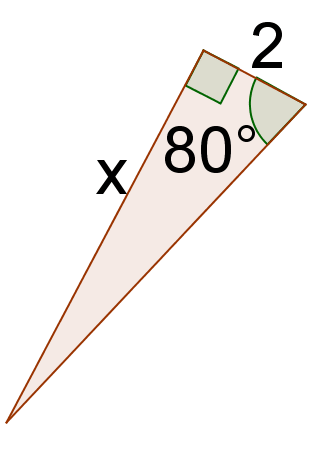# 2.1.7: TAN

•• Contributed by CK12
• CK12

Explore the tangent ratio and use it to find missing sides of right triangles.

As the measure of an angle increases between $$0^{\circ}$$ and $$90^{\circ}$$, how does the tangent ratio of the angle change?

## Tangent Ratio

Recall that one way to show that two triangles are similar is to show that they have two pairs of congruent angles. This means that two right triangles will be similar if they have one pair of congruent non-right angles.Figure $$\PageIndex{1}$$

The two right triangles above are similar because they have two pairs of congruent angles. This means that their corresponding sides are proportional. $$\overline{DF}$$ and $$\overline{AC}$$ are corresponding sides because they are both opposite the $$22^{\circ}$$ angle. $$\dfrac{DF}{AC}=\dfrac{4}{2}=2$$, so the scale factor between the two triangles is 2. This means that $$x=10$$, because $$\dfrac{FE}{CB}=\dfrac{10}{5}=2$$.

The ratio between the two legs of any $$22^{\circ}$$ right triangle will always be the same, because all $$22^{\circ}$$ right triangles are similar. The ratio of the length of the leg opposite the $$22^{\circ}$$ angle to the length of the leg adjacent to the $$22^{\circ}$$ angle will be $$\dfrac{2}{5}=0.4$$. You can use this fact to find a missing side of another $$22^{\circ}$$ right triangle.Figure $$\PageIndex{2}$$

Because this is a $$22^{\circ}$$ right triangle, you know that $$\dfrac{\text{opposite leg}}{\text{adjacent leg}}=\dfrac{2}{5}=0.4$$.

\begin{aligned} \dfrac{\text{opposite leg}}{\text{adjacent leg}}&=0.4 \\ \dfrac{7}{x}&=0.4 \\ 0.4x &=7\\ x&=17.5 \end{aligned}

The ratio between the opposite leg and the adjacent leg for a given angle in a right triangle is called the tangent ratio. Your scientific or graphing calculator has tangent programmed into it, so that you can determine the \dfrac{\text{opposite leg}}{\text{adjacent leg}}\) ratio for any angle within a right triangle. The abbreviation for tangent is tan.

## Calculating Tangent Functions

Use your calculator to find the tangent of $$75^{\circ}$$. What does this value represent?

Make sure your calculator is in degree mode. Then, type “$$\tan(75)$$”.

$$tan(75^{\circ})\approx 3.732$$

This means that the ratio of the length of the opposite leg to the length of the adjacent leg for a $$75^{\circ}$$ angle within a right triangle will be approximately 3.732.Figure $$\PageIndex{3}$$

#### Solving for Unknown Values

1. Solve for $$x$$.Figure $$\PageIndex{4}$$

From the previous problem, you know that the ratio $$\dfrac{\text{opposite leg}}{\text{adjacent leg}} \approx 3.732$$. You can use this to solve for $$x$$.

\begin{aligned} \dfrac{\text { opposite leg }}{\text { adjacent leg }} & \approx 3.732 \\ \dfrac{x}{2} & \approx 3.732 \\ x & \approx 7.464 \end{aligned}

2. Solve for $$x$$ and $$y$$.Figure $$\PageIndex{5}$$

You can use the $$65^{\circ}$$ angle to find the correct ratio between 24 and $$x$$.

\begin{aligned} \tan \left(65^{\circ}\right) &=\dfrac{\text { opposite leg }}{\text { adjacent leg }} \\ 2.145 & \approx \dfrac{24}{x} \\ x & \approx \dfrac{24}{2.145} \\ x & \approx 11.189 \end{aligned}

Note that this answer is only approximate because you rounded the value of $$\tan 65^{\circ}$$. An exact answer will include “tan”. The exact answer is:

$$x=\dfrac{24}{\tan 65^{\circ}}$$

To solve for y, you can use the Pythagorean Theorem because this is a right triangle.

$$\begin{array}{r} 11.189^{2}+24^{2}=y^{2} \\ 701.194=y^{2} \\ 26.48=y \end{array}$$

Example $$\PageIndex{1}$$

Earlier, you were asked how does the tangent ratio of the angle change.

Solution

As the measure of an angle increases between $$0^{\circ}$$ and $$90^{\circ}$$, how does the tangent ratio of the angle change?Figure $$\PageIndex{6}$$

As an angle increases, the length of its opposite leg increases. Therefore, $$\dfrac{\text{opposite leg}}{\text{adjacent leg}}$$ increases and thus the value of the tangent ratio increases.

Example $$\PageIndex{2}$$

Tangent tells you the ratio of the two legs of a right triangle with a given angle. Why does the tangent ratio not work in the same way for non-right triangles?

Solution

Two right triangles with a $$32^{\circ}$$ angle will be similar. Two non-right triangles with a $$32^{\circ}$$ angle will not necessarily be similar. The tangent ratio works for right triangles because all right triangles with a given angle are similar. The tangent ratio doesn't work in the same way for non-right triangles because not all non-right triangles with a given angle are similar. You can only use the tangent ratio for right triangles.

Example $$\PageIndex{3}$$

Use your calculator to find the tangent of $$45^{\circ}$$. What does this value represent? Why does this value make sense?

Solution

$$\tan(45^{\circ})=1$$. This means that the ratio of the length of the opposite leg to the length of the adjacent leg is equal to 1 for right triangles with a $$45^{\circ}$$ angle.Figure $$\PageIndex{7}$$

This should make sense because right triangles with a $$45^{\circ}$$ angle are isosceles. The legs of an isosceles triangle are congruent, so the ratio between them will be 1.

Example $$\PageIndex{4}$$

Solve for $$x$$.Figure $$\PageIndex{8}$$

Solution

Use the tangent ratio of a $$35^{\circ}$$ angle.

\begin{aligned} \tan \left(35^{\circ}\right) &=\dfrac{\text { opposite leg }}{\text { adjacent leg }} \\ \tan \left(35^{\circ}\right) &=\dfrac{x}{18} \\ x &=18 \tan \left(35^{\circ}\right) \\ x & \approx 12.604 \end{aligned}

### Review

1. Why are all right triangles with a $$40^{\circ}$$ angle similar? What does this have to do with the tangent ratio?

2. Find the tangent of $$40^{\circ}$$.

3. Solve for $$x$$.Figure $$\PageIndex{9}$$

4. Find the tangent of $$80^{\circ}$$.

5. Solve for $$x$$.Figure $$\PageIndex{10}$$

6. Find the tangent of $$10^{\circ}$$.

7. Solve for $$x$$.Figure $$\PageIndex{11}$$

9. Find the tangent of $$27^{\circ}$$.

10. Solve for $$x$$.Figure $$\PageIndex{12}$$

11. Find the tangent of $$42^{\circ}$$.

12. Solve for $$x$$.Figure $$\PageIndex{13}$$

13. A right triangle has a $$42^{\circ}$$ angle. The base of the triangle, adjacent to the $$42^{\circ}$$ angle, is 5 inches. Find the area of the triangle.

14. Recall that the ratios between the sides of a 30-60-90 triangle are $$1:\sqrt{3}:2$$. Find the tangent of $$30^{\circ}$$. Explain how this matches the ratios for a 30-60-90 triangle.

15. Explain why it makes sense that the value of the tangent ratio increases as the angle goes from $$0^{\circ}$$ to $$90^{\circ}$$.

## Vocabulary

Term Definition
AA Similarity Postulate If two angles in one triangle are congruent to two angles in another triangle, then the two triangles are similar.
Congruent Congruent figures are identical in size, shape and measure.
Similar Two figures are similar if they have the same shape, but not necessarily the same size.
Tangent The tangent of an angle in a right triangle is a value found by dividing the length of the side opposite the given angle by the length of the side adjacent to the given angle.